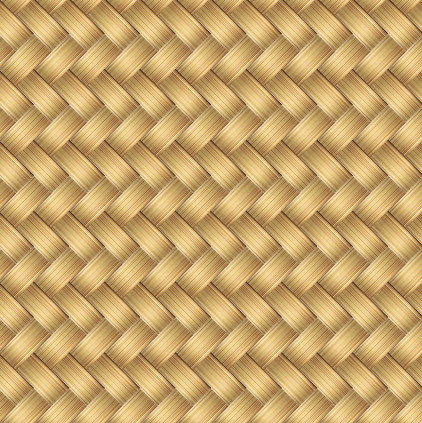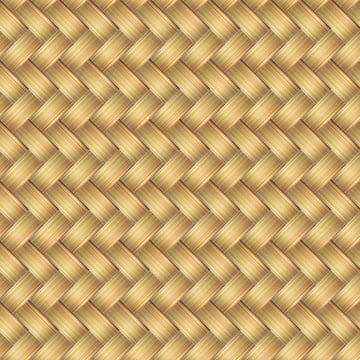# Quick Tip: How to Create a Wicker Seamless Pattern in Adobe IllustratorIn the following tutorial I'm going to show you how to create a wicker seamless pattern in Adobe Illustrator using the Gradient Tool, Blending Modes and the Transform Effect.

## Step 1

Create a New document by hitting Command + N or go to File > New. Enter 600 in the width box and 600 in the height box.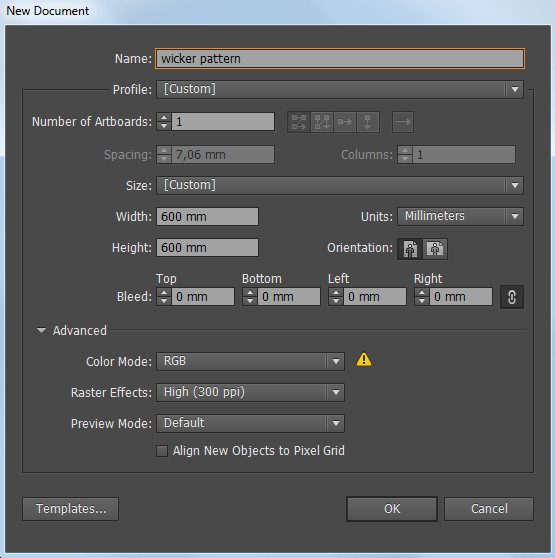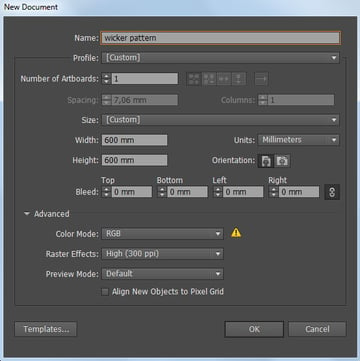## Step 2

Select the Rectangle Tool (M) from the Tool panel and click on the Artboard. The Rectangle Option box will pop up. Set the Width and Height of our basic shape to 100 by 50.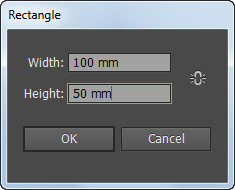## Step 3

Set the Stroke to None. Select the Gradient Tool (G) and use the following colors:

• R=97 G=60 B=42
• R=216 G=166 B=93
• R=255 G=242 B=191
• R=247 G=214 B=132
• R=238 G=214 B=168

Then set the gradient angle to 90°.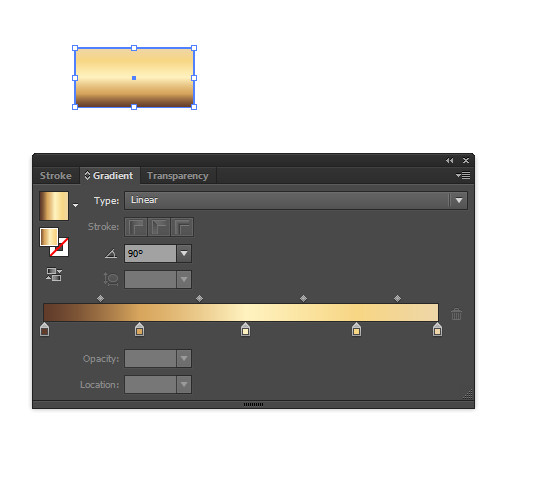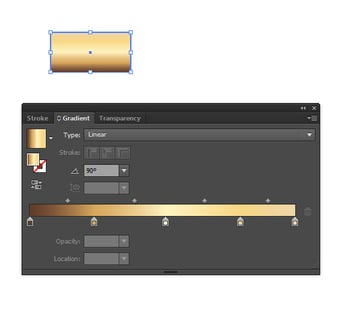Then add some Sliders between the indicated colors and drag them to different positions to achieve the result as mine. Make sure that the colors at the edges are still R=97 G=60 B=42 and R=238 G=214 B=168.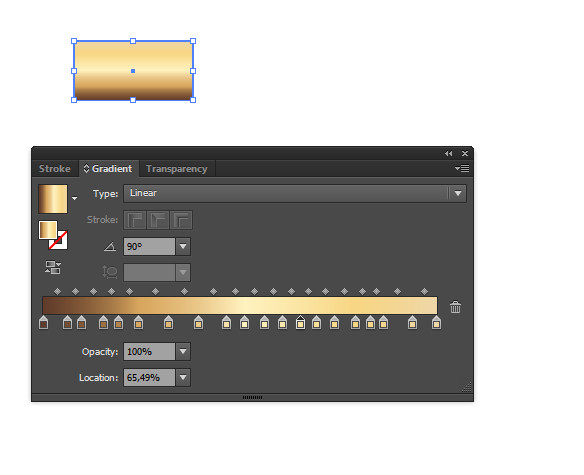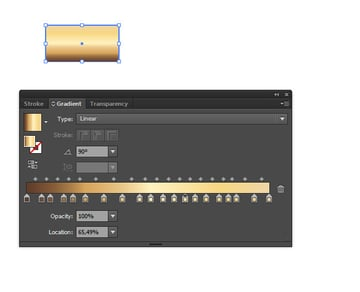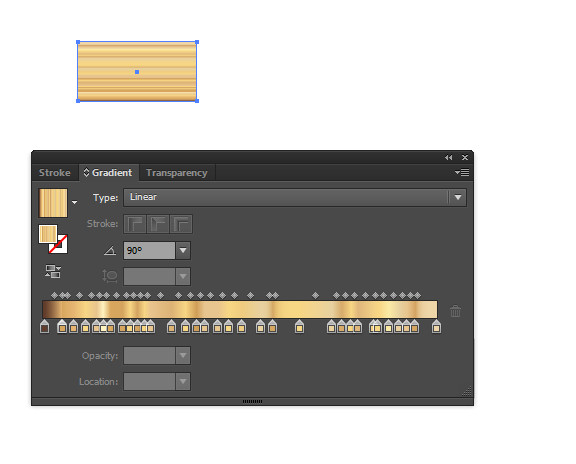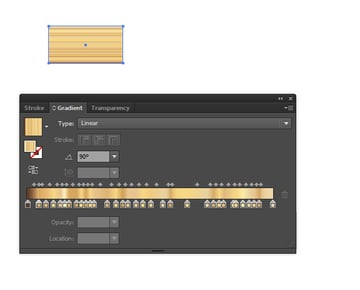## Step 4

Select the created rectangle. Copy it (Command + C) and Paste in Front (Command + F). Fill it with Linear Gradient as shown in the following image. The colors are R=35 G=31 B=32 and R=147 G=149 B=152. Then set the gradient angle to 0°.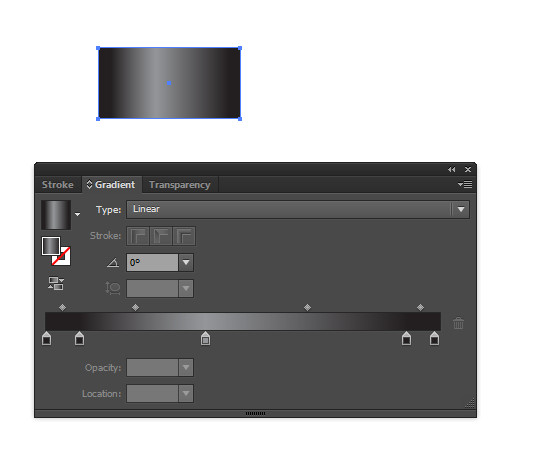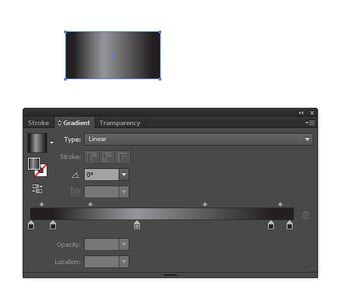## Step 5

Open the Transparency panel (Shift + Command + F10) and change the Blending Mode to Hard Light. Reduce the Opacity to 30%.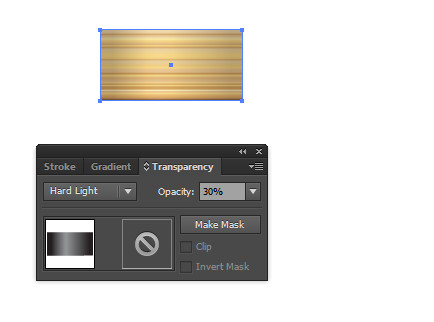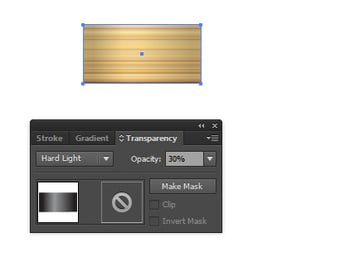## Step 6

Select the rectangle with the brown gradient and Copy it (Command + C) and Paste in Front (Command + F). Change the Blending Mode to Overlay in the Transparency panel. Group these three rectangles together (Command + G).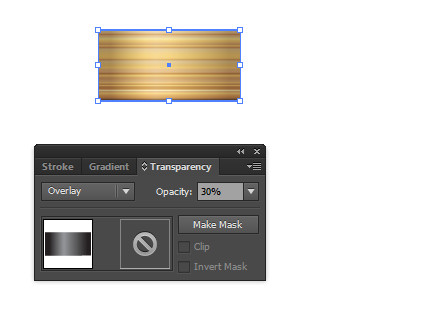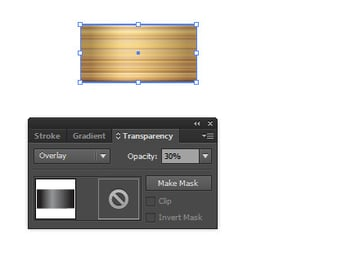## Step 7

With the selected group of rectangles open the Transform panel (Shift + F8) and set the following parameters.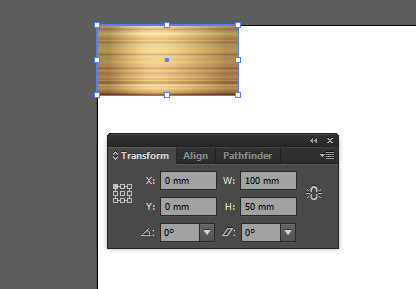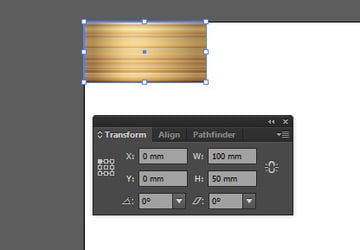## Step 8

Create a copy of the rectangles by pressing Command + C and Command + V. With the new group selected enter 90 in the Rotate field of the Transform panel. Then set the following parameters.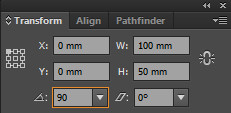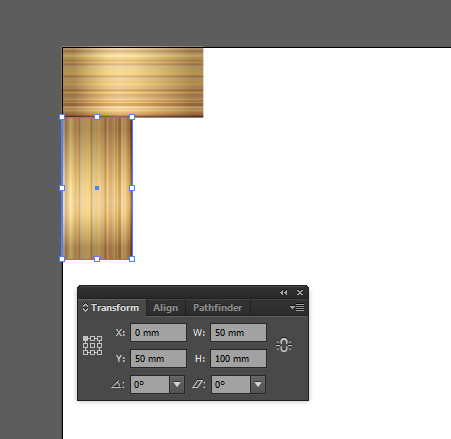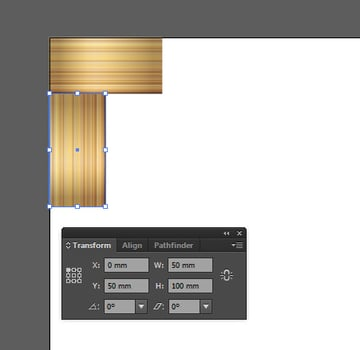## Step 9

Select the both shapes and set 45 in the Rotate field of the Transform panel. Then set the following parameters.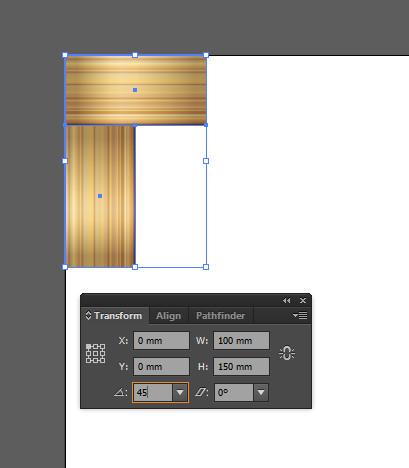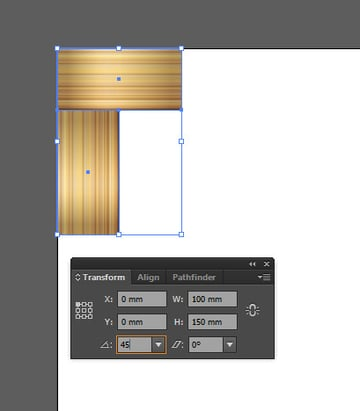## Step 10

With the both rectangles selected change the parameters of Weight and High in the Transform panel as shown in the image below.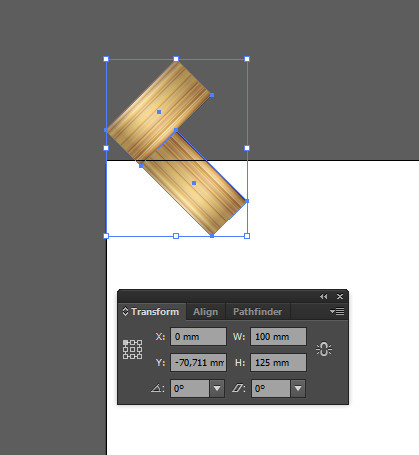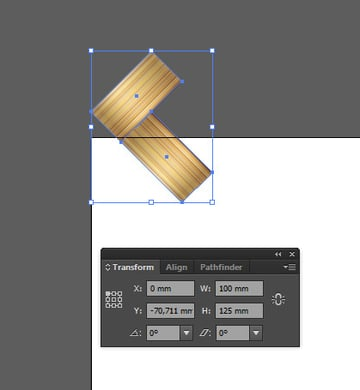## Step 11

Change the position of the rectangles by entering the following values into the X and Y fields of the Transform panel.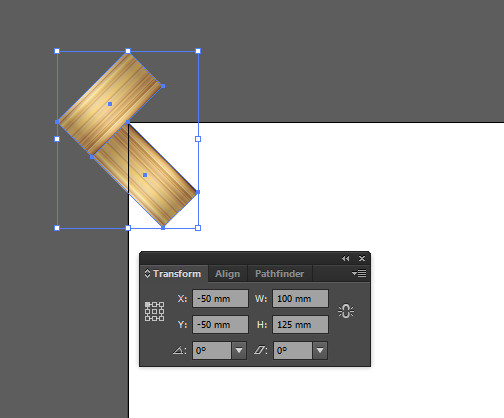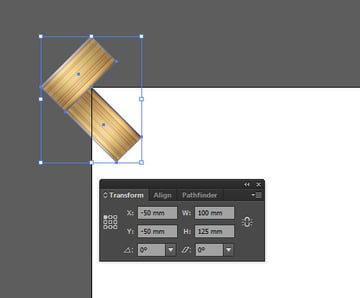## Step 12

With the both rectangles selected go to Effect > Distort & Transform > Transform. Set the following parameters in the Transform Effect window and click OK.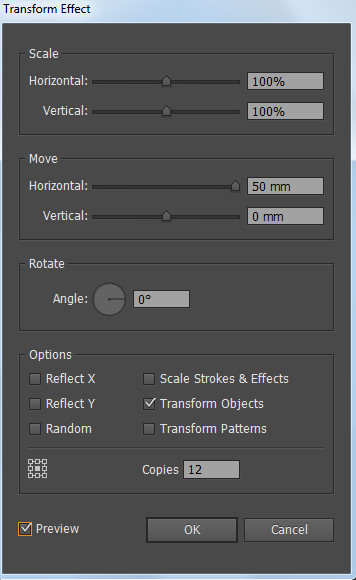We should have the effect as in the image below.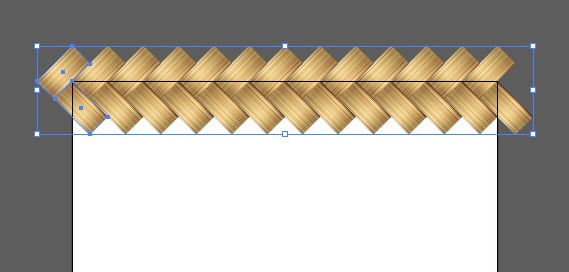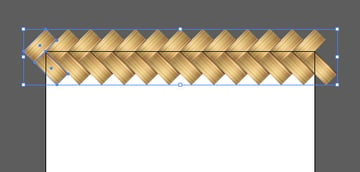## Step 13

Don't remove the selection and go again to Effect > Distort & Transform > Transform. Press Apply New Effect button in the appeared window. Then set the following parameters in the Transform Effect window and click OK.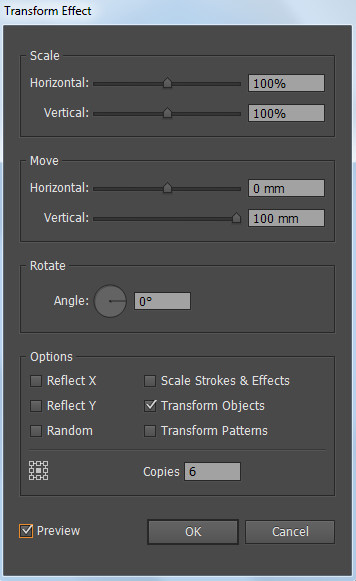We should have the effect as in the image below.

And don't forget to go Object > Expand Appearance.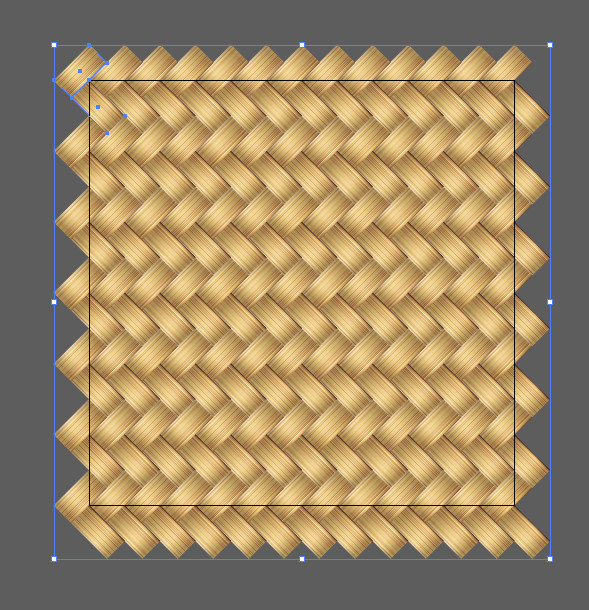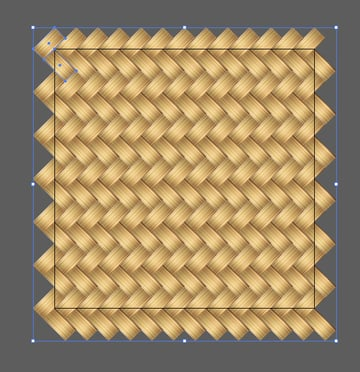## Step 14

Using the Rectangle Tool (M), create a 600 by 600 shape with no Fill and no Stroke. Set 0 in the X and Y fields of the Transform panel. Send the square to the back (Command + Shift + [) and then select all the objects and drag them into the Swatches panel.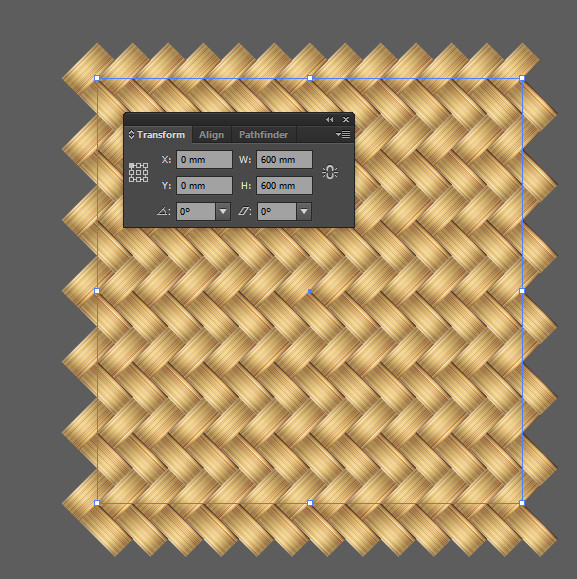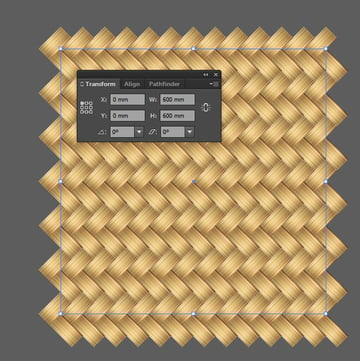## Conclusion

Now we have a wicker seamless pattern. You can create any shape and fill it with the wicker texture from the Swatches panel. Have fun!